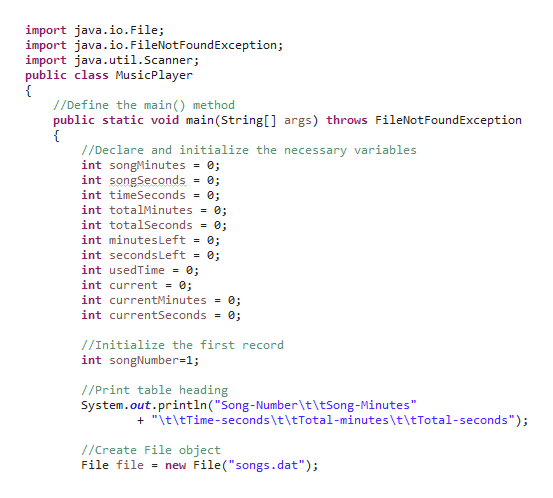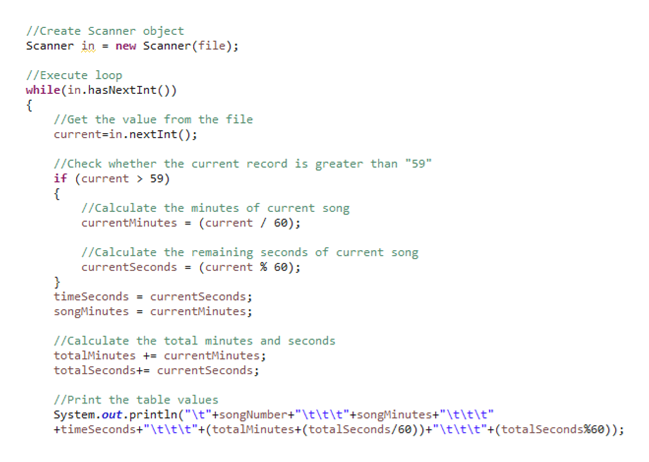# You are burning some music CDs for a party. You've arranged a list of songs in the order in which you want to play them. However, you would like to maximize your use of space on the CD, which holds 80 minutes of music. So, you want to figure out the total time for a group of songs and see how well they fit. Write and design a java program to help you do this. The data are on the file songs.dat. The time is entered as seconds. For example, if a song takes 7 minutes and 42 seconds to play, the data entered in the data file for that songs would be 462.After all the data has been read, the application should print a message indicating the time remaining on the CD.The output should be in the form of a table with columns and headings written on a file. For example:Song Song Time Total TimeNumber Minutes Seconds Minutes Seconds------- ------- ------- ------- -------1 5 10 5 102 7 42 12 523 4 19 17 114 4 33 21 445 10 27 32 116 8 55 41 67 5 0 46 6There are 58 minutes and 54 seconds of space left on the 100 minute CD.Note that the output converts the input from seconds to minutes and seconds. Use meaningful variable names, proper indentation, and appropriate comments. Thoroughly test the program using your own data sets.

Question
84 views

You are burning some music CDs for a party. You've arranged a list of songs in the order in which you want to play them. However, you would like to maximize your use of space on the CD, which holds 80 minutes of music. So, you want to figure out the total time for a group of songs and see how well they fit. Write and design a java program to help you do this. The data are on the file songs.dat. The time is entered as seconds. For example, if a song takes 7 minutes and 42 seconds to play, the data entered in the data file for that songs would be 462.

After all the data has been read, the application should print a message indicating the time remaining on the CD.

The output should be in the form of a table with columns and headings written on a file. For example:

Song Song Time Total Time
Number Minutes Seconds Minutes Seconds
------- ------- ------- ------- -------
1 5 10 5 10
2 7 42 12 52
3 4 19 17 11
4 4 33 21 44
5 10 27 32 11
6 8 55 41 6
7 5 0 46 6

There are 58 minutes and 54 seconds of space left on the 100 minute CD.

Note that the output converts the input from seconds to minutes and seconds. Use meaningful variable names, proper indentation, and appropriate comments. Thoroughly test the program using your own data sets.

check_circle

Step 1

Note: The below program is written for CD which holds 80 minutes of music.

Step 2

A Java program for the given criteria is as follows,

import java.io.File;

import java.io.FileNotFoundException;

import java.util.Scanner;

public class MusicPlayer

{

//Define the main() method

public static void main(String[] args) throws FileNotFoundException

{

//Declare and initialize the necessary variables

int songMinutes = 0;

int songSeconds = 0;

int timeSeconds = 0;

int totalMinutes = 0;

int totalSeconds = 0;

int minutesLeft = 0;

int secondsLeft = 0;

int usedTime = 0;

int current = 0;

int currentMinutes = 0;

int currentSeconds = 0;

//Initialize the first record

int songNumber=1;

System.out.println("Song-Number\t\tSong-Minutes"

+ "\t\tTime-seconds\t\tTotal-minutes\t\tTotal-seconds");

//Create File object

File file = new File("songs.dat");

//Create Scanner object

Scanner in = new Scanner(file);

//Execute loop

while(in.hasNextInt())

{

//Get the value from the file

current=in.nextInt();

//Check whether the current record is greater than "59"

if (current > 59)

{

//Calculate the minutes of current song

currentMinutes = (current / 60);

//Calculate the remaining seconds of current song

currentSeconds = (current % 60);

}

timeSeconds = currentSeconds;

songMinutes = currentMinutes;

//Calculate the total minutes and seconds

totalMinutes += currentMinutes;

totalSeconds+= currentSeconds;

//Print the table values

System.out.println("\t"+songNumber+"\t\t\t"+songMinutes+"\t\t\t"

+timeSeconds+"\t\t\t"+(totalMinutes+(totalSeconds/60))+"\t\t\t"+(totalSeconds%60));

//Calculate the used time

usedTime = (totalMinutes * 60 + totalSeconds);

//Increment the song number

songNumber++;

}

//Calculate the time hold on CD in seconds

int totalTime_CD = 80*60;

//Calculate the minutes left on the CD

minutesLeft = (totalTime_CD - usedTime) / 60;

//Calculate the second left on the Cd

secondsLeft = (totalTime_CD - usedTime) % 60;

//Print the minutes and seconds left on the CD

System.out.println("There are "+minutesLeft+" minutes and "+secondsLeft+

"seconds left of space on the 80 minute CD.");

}

}

Screenshot of program #1:help_outlineImage Transcriptioncloseimport java.io. File; import java.io.FileNot Found Exception; import java.util.Scanner; public class MusicPlayer /Define the main() method public static void main(String [] args) throws FileNotFoundException //Declare and initialize the necessary variables int songMinutes int songSeconds int timeSeconds 0; int totalMinutes= 0; int totalSeconds = 0; int minutes Left = 0; int seconds Left = 0; int usedTime= 0; int current = 0; = =0; int currentMinutes = 0; int currentSeconds = 0; //Initialize the first record int songNumber 1; //Print table heading System.out.println ( "Song - Number\t\tSong -Minutes" "It\tTime-seconds\t\tTotal-minutes\t\tTotal-seconds"); //Create File object File file new File ("songs.dat"); fullscreen
Step 3

Screenshot of program #2...help_outlineImage Transcriptionclose//Create Scanner object Scanner in new Scanner(file ) ; //Execute loop while (in.hasNext Int ( ) ) //Get the value from the file current-in.next Int ( ); //Check whether the current record is greater than "59" if (current 59) //Calculate the minutes of current song currentMinutes- (current / 60); //Calculate the remaining seconds of current song currentSeconds = (current % 60); timeSeconds currentSeconds; songMinutes = currentMinutes; //Calculate the total minutes and seconds totalMinutes += currentMinutes; totalseconds+= currentSeconds; //Print the table values System. out.println ("\t"+songNumber+"\t\t\t "+songMinutes+" \t\t\t"| +timeSeconds+" \t\t\t"+(totalMinutes+(totalSeconds/60) ) +" \t\t\t"+ (totalSeconds%60) ) ; fullscreen

### Want to see the full answer?

See Solution

#### Want to see this answer and more?

Solutions are written by subject experts who are available 24/7. Questions are typically answered within 1 hour.*

See Solution
*Response times may vary by subject and question.
Tagged in

### Java